# In This Exercise We Develop The Annealed Importance Sampling Procedure Assume That W 2528167

In this exercise we develop the annealed importance sampling procedure. Assume that we want to generate samples from a distribution p(x) ∝ f(x) from which sampling is hard. Assume also that we have a distribution q(x) ∝ g(x) from which sampling is easy. In principle, we can use q as a proposal distribution for p, and apply importance sampling. However, if q and p are very different, the results are likely to be quite poor. We now construct a sequence of Markov chains that will allow us to incrementally produce a lower-variance importance-sampling estimator. The technique is as follows. We define a sequence of distributions, p0, . . . , pk, where pi(x) ∝ fi(x), and fi is defined as: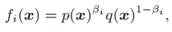where 1 = β0 > β1 > . . . > βk = 0. Note that p’ = p and pk = q. We assume that we can generate samples from pk, and that, for each pi, i = 1, . . . , k − 1, we have a Markov chain Ti whose stationary distribution is pi. To generate a weighted sample x, w relative to our target distribution p, we follow the following algorithm: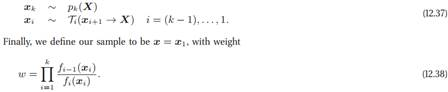To prove that these importance weights are correct, we define both a target distribution and a proposal distribution over the larger state space (x1, . . . , xk). We then show that the importance weights defined in equation (12.38) are correct relative to these distributions over the larger space.

a. Let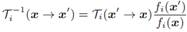define the reversal of the transition model defined by Ti. Show that T−1i (X → X’) is a valid transition model.

b. Define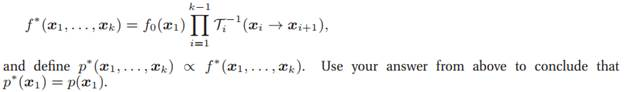c. Let g∗ be the function encoding the joint distribution from which x1, . . . , xk are sampled in the annealed importance sampling procedure equation (12.37). Show that the weight in equation (12.38) can be obtained as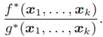One can show, under certain assumptions, that the variance of the weights obtained by this procedure grows linearly in the dimension n of the number of variables X, whereas the variance in a traditional importance sampling procedure grows exponentially in n.